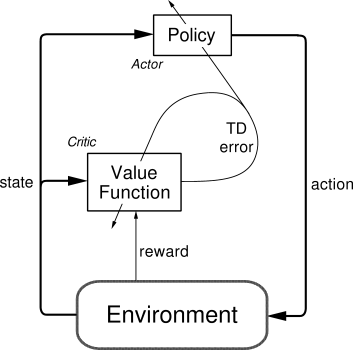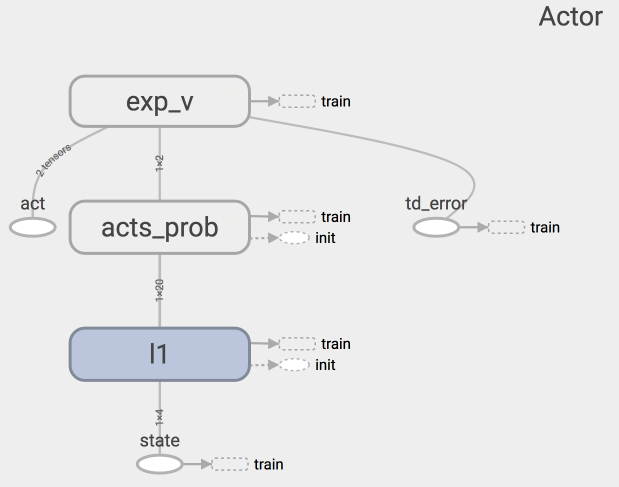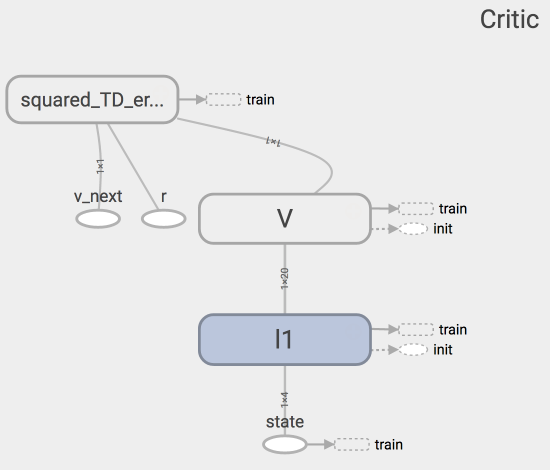# Actor Critic (Tensorflow)

## 要点 ¶

Actor Critic 方法的优势: 可以进行单步更新, 比传统的 Policy Gradient 要快.

Actor Critic 方法的劣势: 取决于 Critic 的价值判断, 但是 Critic 难收敛, 再加上 Actor 的更新, 就更难收敛. 为了解决收敛问题, Google Deepmind 提出了 Actor Critic 升级版 Deep Deterministic Policy Gradient. 后者融合了 DQN 的优势, 解决了收敛难的问题. 我们之后也会要讲到 Deep Deterministic Policy Gradient. 不过那个是要以 Actor Critic 为基础, 懂了 Actor Critic, 后面那个就好懂了.

## 算法 ¶

Actor 修改行为时就像蒙着眼睛一直向前开车, Critic 就是那个扶方向盘改变 Actor 开车方向的.## 代码主结构 ¶class Actor(object):
def __init__(self, sess, n_features, n_actions, lr=0.001):
# 用 tensorflow 建立 Actor 神经网络,
# 搭建好训练的 Graph.

def learn(self, s, a, td):
# s, a 用于产生 Gradient ascent 的方向,
# td 来自 Critic, 用于告诉 Actor 这方向对不对.

def choose_action(self, s):
# 根据 s 选 行为 aclass Critic(object):
def __init__(self, sess, n_features, lr=0.01):
# 用 tensorflow 建立 Critic 神经网络,
# 搭建好训练的 Graph.

def learn(self, s, r, s_):
# 学习 状态的价值 (state value), 不是行为的价值 (action value),
# 计算 TD_error = (r + v_) - v,
# 用 TD_error 评判这一步的行为有没有带来比平时更好的结果,
return # 学习时产生的 TD_error


## 两者学习方式 ¶

Actor 想要最大化期望的 reward, 在 Actor Critic 算法中, 我们用 “比平时好多少” (TD error) 来当做 reward, 所以就是:

with tf.variable_scope('exp_v'):
log_prob = tf.log(self.acts_prob[0, self.a])    # log 动作概率
self.exp_v = tf.reduce_mean(log_prob * self.td_error)   # log 概率 * TD 方向
with tf.variable_scope('train'):
# 因为我们想不断增加这个 exp_v (动作带来的额外价值),
# 所以我们用过 minimize(-exp_v) 的方式达到
# maximize(exp_v) 的目的


Critic 的更新很简单, 就是像 Q learning 那样更新现实和估计的误差 (TD error) 就好了.

with tf.variable_scope('squared_TD_error'):
self.td_error = self.r + GAMMA * self.v_ - self.v
self.loss = tf.square(self.td_error)    # TD_error = (r+gamma*V_next) - V_eval
with tf.variable_scope('train'):


## 每回合算法 ¶

for i_episode in range(MAX_EPISODE):
s = env.reset()
t = 0
track_r = []    # 每回合的所有奖励
while True:
if RENDER: env.render()

a = actor.choose_action(s)

s_, r, done, info = env.step(a)

if done: r = -20    # 回合结束的惩罚

track_r.append(r)

td_error = critic.learn(s, r, s_)  # Critic 学习
actor.learn(s, a, td_error)     # Actor 学习

s = s_
t += 1

if done or t >= MAX_EP_STEPS:
# 回合结束, 打印回合累积奖励
ep_rs_sum = sum(track_r)
if 'running_reward' not in globals():
running_reward = ep_rs_sum
else:
running_reward = running_reward * 0.95 + ep_rs_sum * 0.05
if running_reward > DISPLAY_REWARD_THRESHOLD: RENDER = True  # rendering
print("episode:", i_episode, "  reward:", int(running_reward))
break